# Statistical simulation of robust estimators with tidyverse tools

Functions from the tidyverse provide a powerful way to do statistical simulations. We demonstrate this approach by evaluating the properties of the mean and median as estimators of center for two distributions.”
Author

Max Rohde

Published

January 13, 2021

Code
# Load packages
library(tidyverse)

# ggplot2 themes
library(cowplot)

# Formatting of HTML tables
library(kableExtra)

set.seed(7)

The sample mean and sample median are commonly used estimators for the center of distribution. There is no such thing as a “best estimator” in all circumstances. However, estimators can definitely outperform other estimators in terms of desirable properties (e.g., unbiasedness, low variance, consistency) given a particular circumstance. We can use simulation and mathematical theory to evaluate to performance of estimators given a set of assumptions. Here we focus on using simulation, with the help of tools from the tidyverse.

## Scenario 1

We begin with a simple example. The true data generating process is

$X_1, X_2, \ldots X_n \stackrel{iid}{\sim} N(3,1)$

How will the mean and median perform as estimators of center? Let’s use simulation to find out.

We will use a tibble to store all of our simulation results. First, let’s decide what sample sizes to simulate, and how many trials to run. The more trials we run, the more accurate our simulation results will be – the only cost being increased time to run the simulations.

Code
size <- c(5,10,20,50,100,200)
trial <- 1:1e5

Now we use crossing() to generate a tibble that contains every combination of the vectors size and trial. So for every sample size, we are repeating it 100,000 times.

Code
df <- crossing(trial, size)
# A tibble: 600,000 × 2
trial  size
<int> <dbl>
1     1     5
2     1    10
3     1    20
4     1    50
5     1   100
6     1   200
7     2     5
8     2    10
9     2    20
10     2    50
# … with 599,990 more rows

Now in each row, we want a unique sample of data with the sample size given by that row. We will use purrr::map() to do this.

Code
df$data <- map(df$size, ~rnorm(n=.x, mean = 3, sd=1))

The first argument to map is the vector to iterate over, and the second argument is the function to apply. We use .x as a dummy variable to refer to the value in the current iteration. In words, we are mapping each sample size to a random sample of size $$n$$ from a normal distribution.

The new column, data, is a vector of lists, where each list contains a unique sample of data. Let’s see what this looks like.

# A tibble: 600,000 × 3
trial  size data
<int> <dbl> <list>
1     1     5 <dbl >
2     1    10 <dbl >
3     1    20 <dbl >
4     1    50 <dbl >
5     1   100 <dbl >
6     1   200 <dbl >
7     2     5 <dbl >
8     2    10 <dbl >
9     2    20 <dbl >
10     2    50 <dbl >
# … with 599,990 more rows

Now that we have our data, we can compute the mean and median for each sample.

Code
df$mean <- map_dbl(df$data, ~mean(.x))
df$median <- map_dbl(df$data, ~median(.x))
# A tibble: 600,000 × 5
trial  size data         mean median
<int> <dbl> <list>      <dbl>  <dbl>
1     1     5 <dbl >    2.80   2.31
2     1    10 <dbl >   3.96   3.55
3     1    20 <dbl >   3.14   3.15
4     1    50 <dbl >   3.07   3.09
5     1   100 <dbl >  3.16   3.32
6     1   200 <dbl >  2.96   2.95
7     2     5 <dbl >    2.52   2.78
8     2    10 <dbl >   2.83   2.80
9     2    20 <dbl >   3.08   3.14
10     2    50 <dbl >   2.93   2.86
# … with 599,990 more rows

The mean and median of each sample are now in separate columns. However, to get the data into tidy format, also known as long format, we want them in separate rows. Having the data in tidy format allows us to use ggplot2 and other tidyverse functions more effectively. We use pivot_longer to do this.

Code
df <- pivot_longer(df, cols=mean:median, names_to="Estimator", values_to="Estimate")
# A tibble: 1,200,000 × 5
trial  size data        Estimator Estimate
<int> <dbl> <list>      <chr>        <dbl>
1     1     5 <dbl >   mean          2.80
2     1     5 <dbl >   median        2.31
3     1    10 <dbl >  mean          3.96
4     1    10 <dbl >  median        3.55
5     1    20 <dbl >  mean          3.14
6     1    20 <dbl >  median        3.15
7     1    50 <dbl >  mean          3.07
8     1    50 <dbl >  median        3.09
9     1   100 <dbl > mean          3.16
10     1   100 <dbl > median        3.32
# … with 1,199,990 more rows

Now we are finally ready to analyze the results of our simulation. First, let’s compute the bias and variance of our estimators for each sample size.

Code
df %>%
group_by(size, Estimator) %>%
summarize(Bias = (mean(Estimate) - 3),
Variance = var(Estimate)) %>%
pivot_longer(Bias:Variance) -> summary_df
# A tibble: 24 × 4
# Groups:   size 
size Estimator name          value
<dbl> <chr>     <chr>         <dbl>
1     5 mean      Bias      0.0000212
2     5 mean      Variance  0.199
3     5 median    Bias      0.00141
4     5 median    Variance  0.286
5    10 mean      Bias     -0.000959
6    10 mean      Variance  0.100
7    10 median    Bias     -0.000314
8    10 median    Variance  0.138
9    20 mean      Bias      0.000634
10    20 mean      Variance  0.0502
# … with 14 more rows

Plotting the bias and variance as a function of sample size, we see that both the mean and median are unbiased estimators of the center of the true distribution, but the median has higher variance. Therefore, we would prefer the mean under these assumptions.

Code
summary_df %>%
ggplot() +
aes(x=size, y=value, color=Estimator) +
geom_line(alpha=0.6) +
geom_point(alpha=0.6) +
facet_wrap(~name) +
scale_color_brewer(palette = "Set1") +
cowplot::theme_cowplot(font_size = 12, font_family = "Lato") +
theme(legend.position = c(0.8,0.8)) +
labs(
x = "Sample Size",
y = "Estimated Value"
)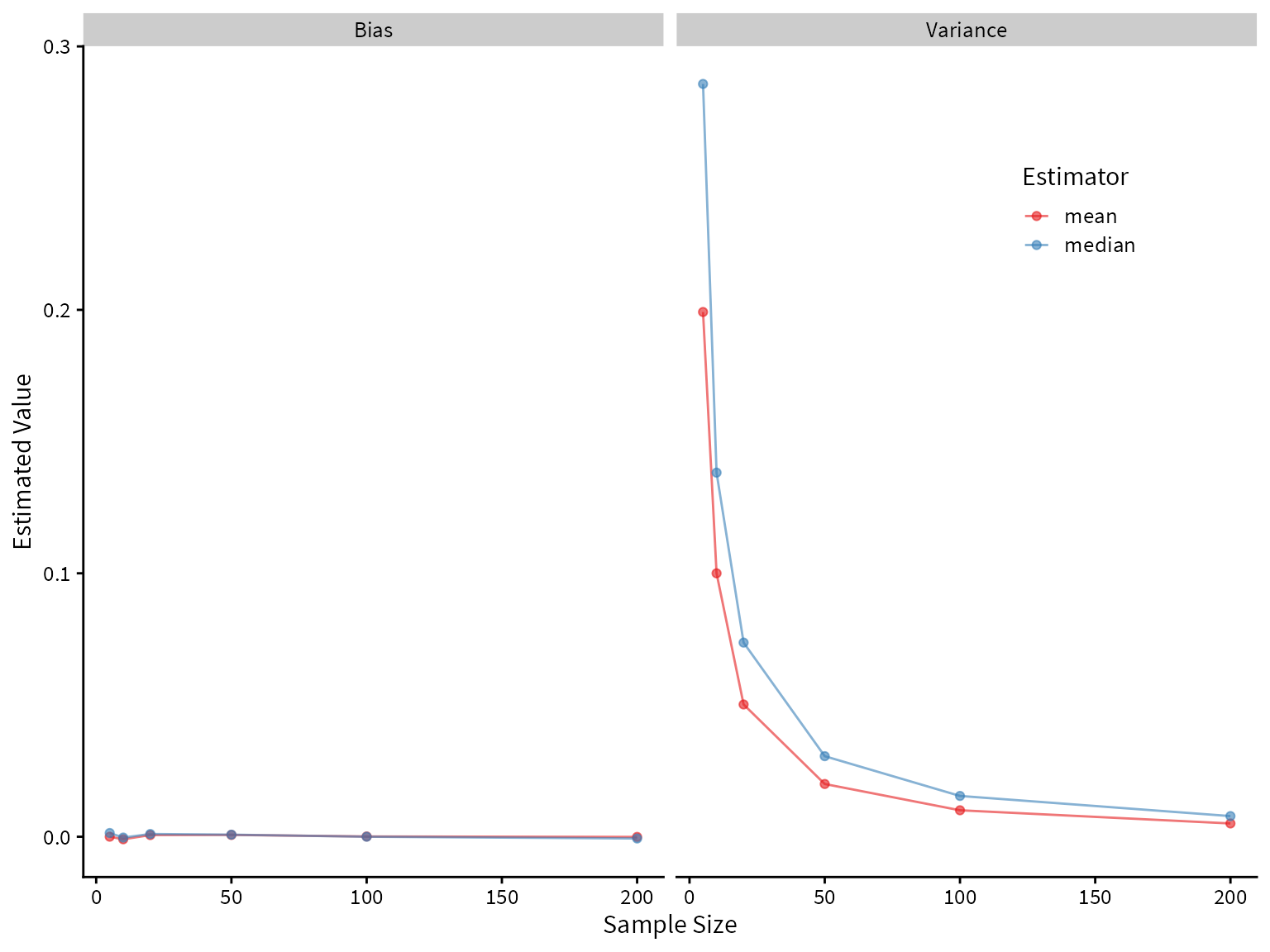Plotting the sampling distribution for each of the estimators shows that the median indeed has higher variance.

Code
label_names <- as_labeller(c(5 = "Sample Size: 5",
10 = "Sample Size: 10",
20 = "Sample Size: 20",
50 = "Sample Size: 50",
100 = "Sample Size: 100",
200 = "Sample Size: 200"))

df %>%
ggplot() +
aes(x=Estimate, color=Estimator, fill=Estimator) +
geom_density(alpha=0.3, size=0.8) +
facet_wrap(~size, labeller=label_names) +
geom_vline(aes(xintercept = 3), linetype=2, alpha=0.3) +
coord_cartesian(xlim=c(1,5), ylim=c(0,6)) +
cowplot::theme_cowplot(font_family = "Lato", font_size=12) +
scale_fill_brewer(palette = "Set1") +
scale_color_brewer(palette = "Set1") +
theme(legend.position = c(0.88,0.88)) +
labs(
title = "Normal(3,1) Distribution",
subtitle = "Performance of mean and median",
x="Estimate",
y="PDF"
)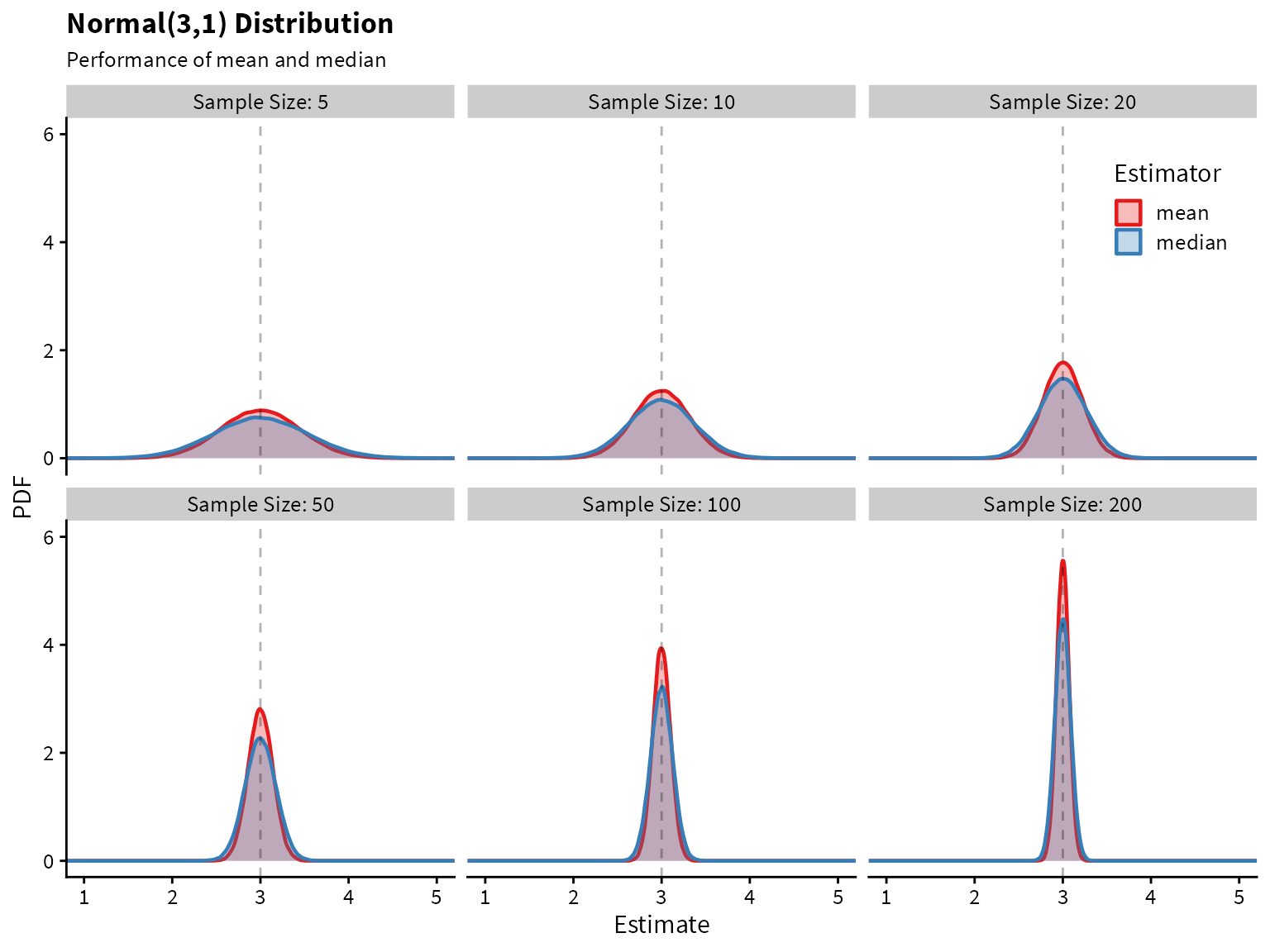## Scenario 2

Now let’s take a look at a distribution with heavier tails than the normal. An example is a mixture of normal two distributions.

The data-generating process is this: With probability 0.9, draw from the $$N(3, 1)$$ distribution. Otherwise, (with probability 0.1), draw from the $$N(3, 10)$$ distribution.

We can write a function to draw from this distribution.

Code
# generates 1 draw from the specifies mixture normal distribution
mixed_normal <- function(){
x <- runif(1)
if (x>0.1) {
return(rnorm(n=1, mean = 3, sd=1))
}
else{
return(rnorm(n=1, mean = 3, sd=10))
}
}

# generates n draws from the specifies mixture normal distribution
rmixed_norm  <- function(n){
map_dbl(1:n, ~mixed_normal())
}

Plotting the normal distribution and the mixture distribution on top of each other, we see that they are very similar, but the mixture distribution has heavier tails (i.e., more of the probability mass is in the tails compared to the normal distribution).

Code
tibble(normal = rnorm(1e5, mean=3),
mixture = rmixed_norm(1e5)) %>%
pivot_longer(cols=normal:mixture, names_to="Distribution", values_to="value") %>%
ggplot() +
aes(x=value, color=Distribution) +
geom_density(alpha=0.7)+
cowplot::theme_cowplot(font_family = "Source Sans Pro", font_size=12) +
scale_color_brewer(palette = "Set1") +
theme(legend.position = c(0.88,0.90)) +
labs(
title = "Mixture Normal vs Normal Distribution",
subtitle = "",
x= "X",
y= "PDF"
)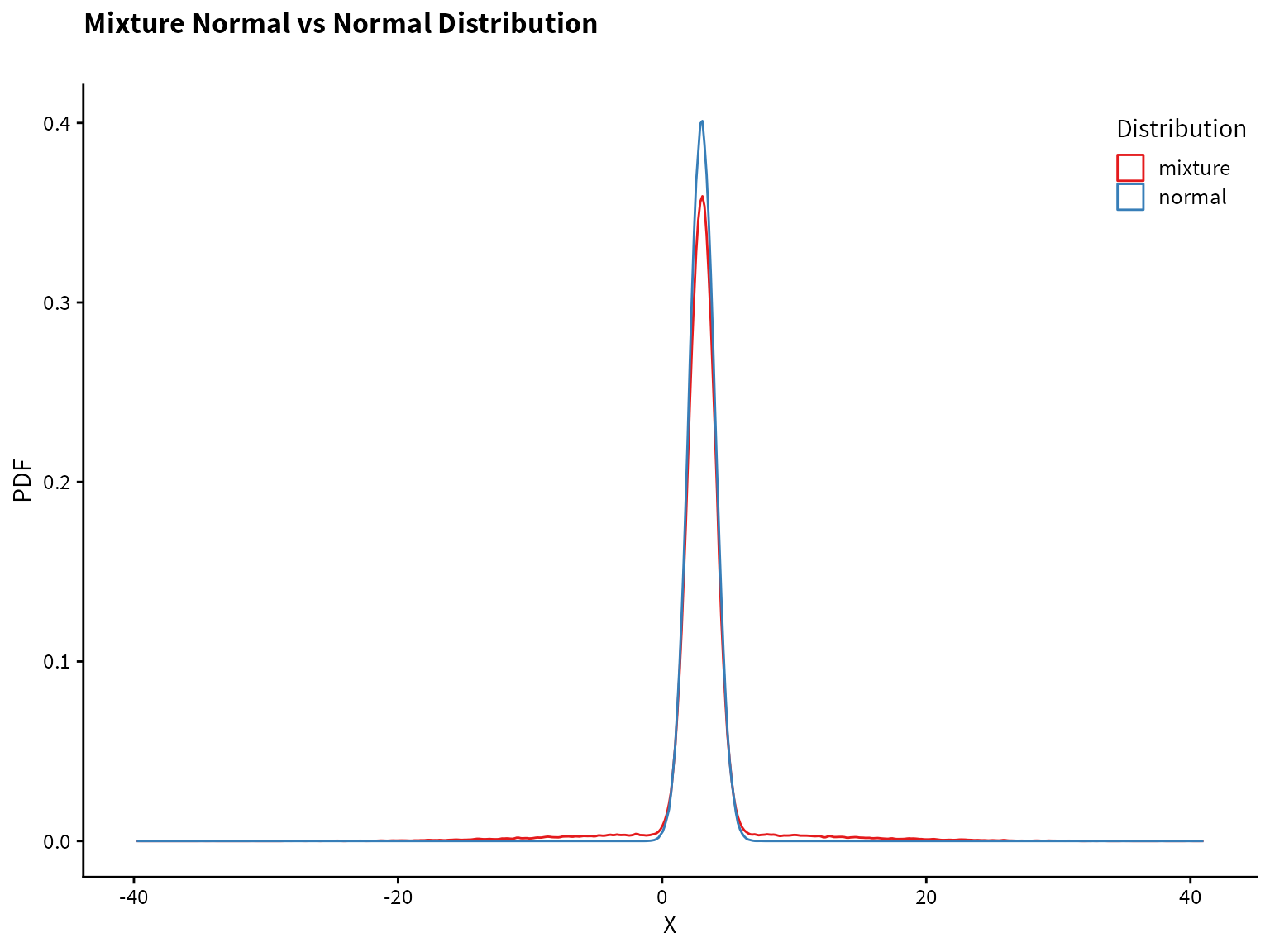Now let’s compare the performance of the mean and median on the mixture distribution.

Code
size <- c(5,10,20,50,100,200)
trial <- 1:1e5

df <- crossing(trial, size)

df$data <- map(df$size, ~rmixed_norm(n=.x))

df$mean <- map_dbl(df$data, ~mean(.x))
df$median <- map_dbl(df$data, ~median(.x))

df <- pivot_longer(df, cols=mean:median, names_to="Estimator", values_to="Estimate")

df %>%
group_by(size, Estimator) %>%
summarize(Bias = (mean(Estimate) - 3),
Variance = var(Estimate)) %>%
pivot_longer(Bias:Variance) -> summary_df

summary_df %>%
ggplot() +
aes(x=size, y=value, color=Estimator) +
geom_line(alpha=0.6) +
geom_point(alpha=0.6) +
facet_wrap(~name) +
scale_color_brewer(palette = "Set1") +
cowplot::theme_cowplot(font_size = 12, font_family = "Lato") +
theme(legend.position = c(0.8,0.8)) +
labs(
x = "Sample Size",
y = "Estimated Value"
)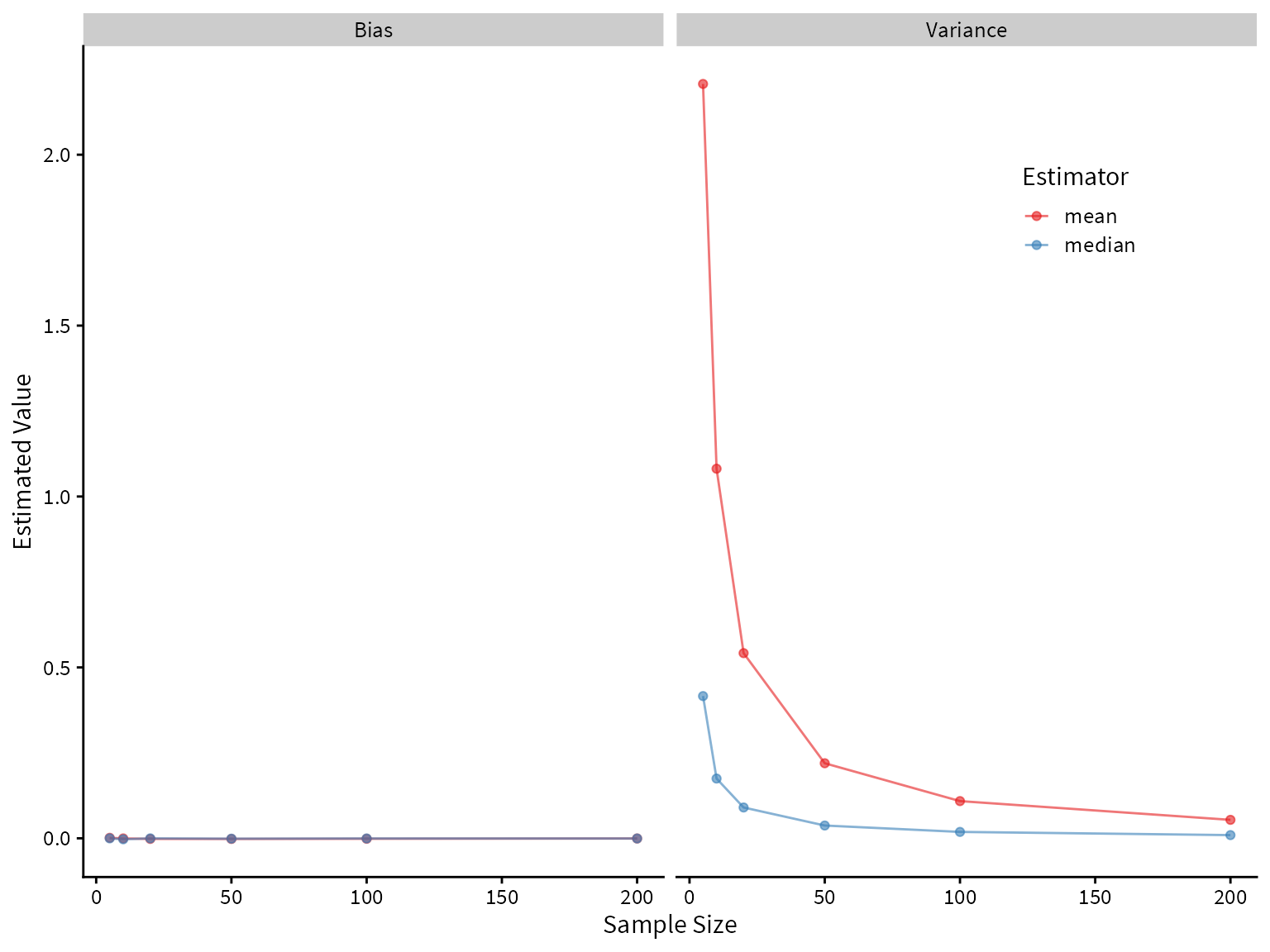Code
label_names <- as_labeller(c(5 = "Sample Size: 5",
10 = "Sample Size: 10",
20 = "Sample Size: 20",
50 = "Sample Size: 50",
100 = "Sample Size: 100",
200 = "Sample Size: 200"))

df %>%
ggplot() +
aes(x=Estimate, color=Estimator, fill=Estimator) +
geom_density(alpha=0.3, size=0.8) +
facet_wrap(~size, labeller=label_names) +
geom_vline(aes(xintercept = 3), linetype=2, alpha=0.3) +
coord_cartesian(xlim=c(1,5), ylim=c(0,6)) +
cowplot::theme_cowplot(font_family = "Lato", font_size=12) +
scale_fill_brewer(palette = "Set1") +
scale_color_brewer(palette = "Set1") +
theme(legend.position = c(0.88,0.88)) +
labs(
title = "Mixture Normal Distribution",
subtitle = "Performance of mean and median",
x="Estimate",
y="PDF"
)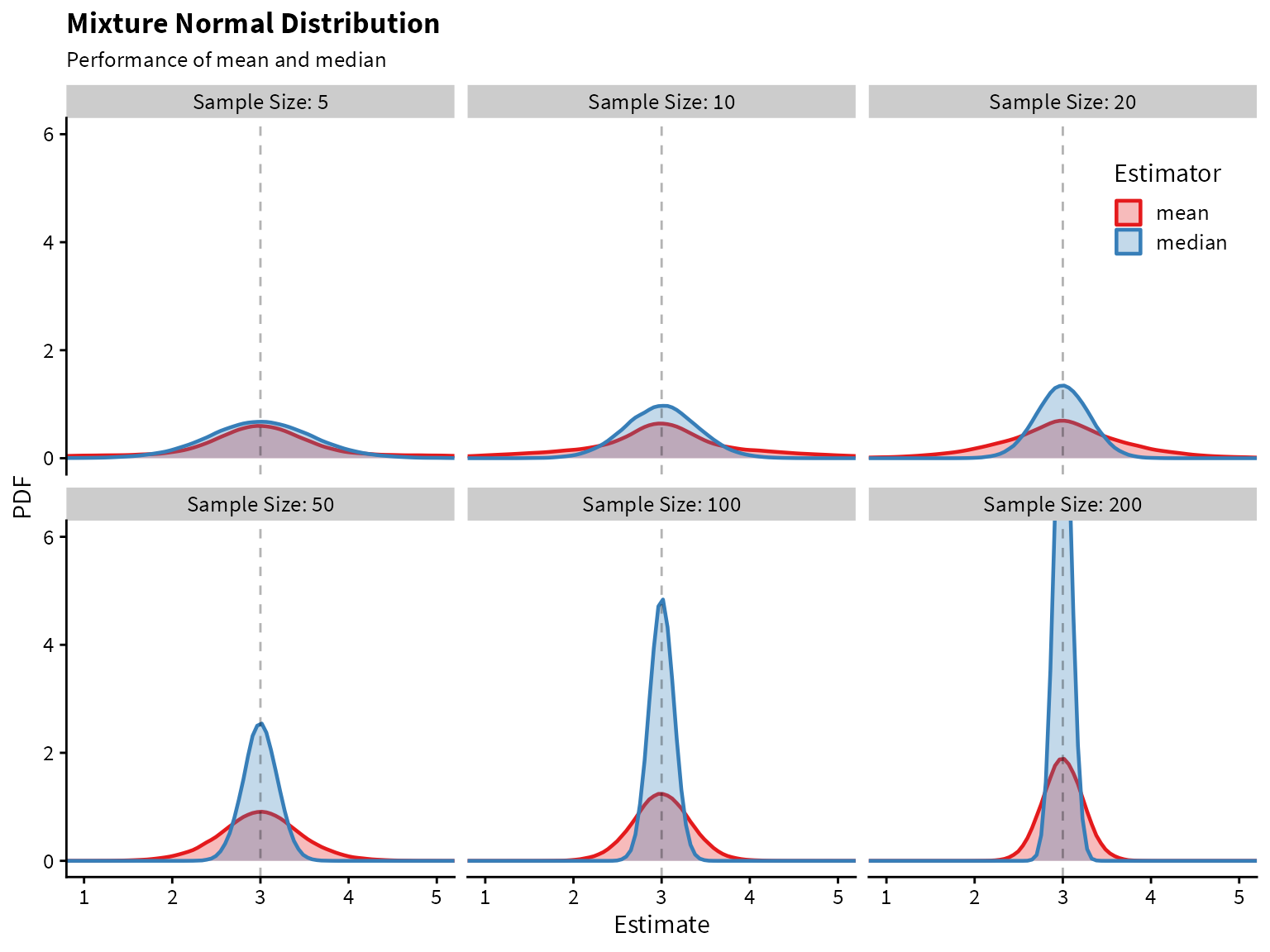It looks like the median greatly outperforms the mean! Both are unbiased, but the median has lower variance.

## Take-away points

• Simulation is a powerful tool in statistics. Here we showed how is can be used to compare the properties of estimators.
• For distributions with heavy tails, the median may be a better estimator of center than the mean.